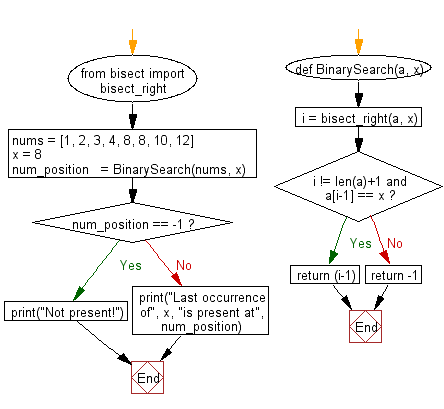﻿ Python Bisect: Find the index position of the last occurrence of a given number in a sorted list using Binary Search - w3resource# Python Bisect: Find the index position of the last occurrence of a given number in a sorted list using Binary Search

## Python Bisect: Exercise-6 with Solution

Write a Python program to find the index position of the last occurrence of a given number in a sorted list using Binary Search (bisect).

Sample Solution:

Python Code:

``````from bisect import bisect_right
def BinarySearch(a, x):
i = bisect_right(a, x)
if i != len(a)+1 and a[i-1] == x:
return (i-1)
else:
return -1
nums = [1, 2, 3, 4, 8, 8, 10, 12]
x = 8
num_position   = BinarySearch(nums, x)
if num_position == -1:
print("not presetn!")
else:
print("Last occurrence of", x, "is present at", num_position)
```
```

Sample Output:

```Last occurrence of 8 is present at 5
```

Flowchart:## Visualize Python code execution:

The following tool visualize what the computer is doing step-by-step as it executes the said program:

Python Code Editor:

Next: Write a Python program to find three integers which gives the sum of zero in a given array of integers using Binary Search (bisect).

What is the difficulty level of this exercise?

Test your Programming skills with w3resource's quiz.

﻿

## Python: Tips of the Day

How to make a chain of function decorators?

```from functools import wraps

def makebold(fn):
@wraps(fn)
def wrapped(*args, **kwargs):
return "<b>" + fn(*args, **kwargs) + "</b>"
return wrapped

def makeitalic(fn):
@wraps(fn)
def wrapped(*args, **kwargs):
return "<i>" + fn(*args, **kwargs) + "</i>"
return wrapped

@makebold
@makeitalic
def hello():
return "hello world"

@makebold
@makeitalic
def log(s):
return s

print hello()        # returns "<b><i>hello world</i></b>"
print hello.__name__ # with functools.wraps() this returns "hello"
print log('hello')   # returns "<b><i>hello</i></b>"
```

Ref: https://bit.ly/3cVz5iw